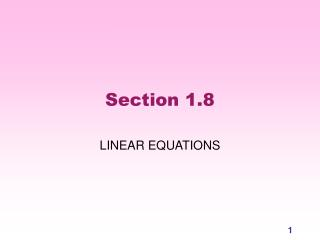Download PresentationSection 1.8

Loading in 2 Seconds...

# Section 1.8 - PowerPoint PPT Presentation

Section 1.8. LINEAR EQUATIONS. Introduction. First, take a few minutes to read the introduction on p. 112. What is the only technique we have learned so far for actually solving DEs? Why aren’t you going to learn how to solve every DE in this course? (Is Dr. Hall being lazy?)I am the owner, or an agent authorized to act on behalf of the owner, of the copyrighted work described.
Download Presentation## Section 1.8

An Image/Link below is provided (as is) to download presentation

Download Policy: Content on the Website is provided to you AS IS for your information and personal use and may not be sold / licensed / shared on other websites without getting consent from its author.While downloading, if for some reason you are not able to download a presentation, the publisher may have deleted the file from their server.

- - - - - - - - - - - - - - - - - - - - - - - - - - E N D - - - - - - - - - - - - - - - - - - - - - - - - - -
Presentation Transcript
1. Section 1.8 LINEAR EQUATIONS

2. Introduction First, take a few minutes to read the introduction on p. 112. • What is the only technique we have learned so far for actually solving DEs? • Why aren’t you going to learn how to solve every DE in this course? (Is Dr. Hall being lazy?) • What tools and techniques are available if you don’t know how to solve a DE? Which of them will give you exact solutions?

3. Definition A first-order* differential equation is linear if it can be written in the form where a(t) and b(t) are any functions of t. Examples: NOT linear: Find a(t) and b(t). Explain why this isn’t linear. *First order means that the equation contains only first derivatives (dy/dt, not d2y/dt2).

4. Read p. 114, top. Choose all that apply: dy/dt = 3y - 7 dy/dt = cost + y2 dy/dt = yet dy/dt = 0 dy/dt = t2(1 + y) linear homogeneous linear nonhomogeneous linear constant-coefficient none of the above Match-making!

5. Answers A23, B4, C1, D13, E2

6. The homogeneous case • The linearity principle: If yh(t) is a solution of a linear homogenous DE, then so is any constant multiple of yh(t). Explain why this is true. • What technique can you use to solve a linear homogeneous DE? Explain. • Solve

7. The nonhomogeneous case Suppose yh(t) is a solution of the homogeneous DE and suppose yp(t) is a particular solution of the nonhomogeneous DE Let y(t) = yh(t)+yp(t). Show that y(t) is a solution of the nonhomogeneous DE above. Hint: plug y(t) into the nonhomogeneous equation.

8. YOU make the call! A tale of two equations: (NDE) which is a nonhomogeneous DE (assume b(t)≠0), and (AHDE) which is its associated homogeneous DE. Make a hypothesis about the relationship between solutions of (NDE) and (AHDE). Check your hypothesis by plugging into (NDE). Try to make your hypothesis as general as possible. Hint: use the linearity principle if you haven’t already.

9. Solving Linear Equations Three-step procedure: • Find the general solution of the associated homogeneous DE. • Find one particular solution of the nonhomogeneous DE. • The general solution of the nonhomogeneous DE is the sum of the solutions you found in steps (1) and (2).

10. Arg! Step (2) is tricky We haven’t developed any general techniques for finding even one solution of a nonhomogeneous DE. Hmmm… Maybe we could just, like, guess a solution. Maybe we’d get lucky! After all, we only need one. This extremely precise scientific technique is known as the METHOD OF LUCKY GUESS. (To tell the truth, a lot of math and science works this way: you have a hunch that something is true, then you try to prove (or disprove) it.)

11. The lucky guess Read p. 118. • Write down the NDE, its AHDE, and the general solution to the AHDE. • What initial guess do the authors make? • Why is it not right? • What is their next guess? • Explain how they finish the problem. • Guess a particular solution to p. 123 #1. • Did you get lucky? • Finish the problem.

12. Another lucky guess Read p. 119 • Why do they guess a sine plus a cosine, rather than just a sine or a cosine? Try yp(t) = cos 3t if you’re not convinced. • Explain each step in the computation. In particular, explain where the “simultaneous algebraic equations” come from. • What guesses should you make for p. 123, #3 and #4? • Exercise: do p. 123 #3 • The method we just developed is called The Method of Undetermined Coefficients ( and  are the undetermined coefficients).

13. Qualitative analysis • Use the HPGSolver to analyze solutions to qualitatively. What is the long-term behavior of every solution? • Read p. 120-1 for an explanation of this. • Now tell me about the long-term behavior of solutions to a) and b)